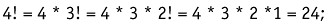# Recursion

Recursion is a programming technique where one function calls itself to iteratively process a problem (Figure 9.1).

##### Figure 9.1. Flowchart of the Recursive Process## R ECURSION

If you don't get it, see recursion.

It is important to note that each recursion must depend on the result of a conditional test. If not, we would end up with infinite recursion, a similar problem to an infinite loop.

## I NFINITE R ECURSION

See infinite recursion.

Suppose we create a factorical function. The factorial of a number is the product of every positive nonzero integer less than or equal to itself. So, for instance, 4! (four factorial):Through a little reworking we see that the general solution is x * ( x “ 1)! .The recursive potential should be clear.

##### ActionScript
` factorial (x) {    return  (x==1)?   1   :    x * factorial (x-1); } `

Recursive functions and loops are very similar. Theoretically, anything that can be written using one can be written using the other. But usually one solution is the natural choice. Churning through a list is easily handled by a for loop . Calculating a factorial is naturally a recursive function.

To get more technical, the major difference in the execution of a loop and a recursive function has to do with the stack. An advanced discussion of the stack is beyond the scope of the book, but some familiarity is required to understand the difference between calls and loops.

The stack stores the current state immediately prior to a function call. Consider these two implications. First, when the recursive function returns, the calling function continues through the codeblock from where it was called with the original values (except what the called function returns). By contrast, variables of loops do not revert to their original values. Second, a stack overflow error can occur. While a loop can go through nigh-infinite cycles fairly easily, every time a recursive function calls itself the stack grows. After the stack gets too large, errors occur and programs crash.

Tree-walking (following all the members of all the generations of a hierarchical structure) is a nasty process using linear looping techniques. Just look at the nasty explicit addressing in our previous code. It is ugly and still performs only a limited job.

Suddenly, in the recursive solution, all is clean.Flash and XML[c] A Developer[ap]s Guide
ISBN: 201729202
EAN: N/A
Year: 2005
Pages: 160